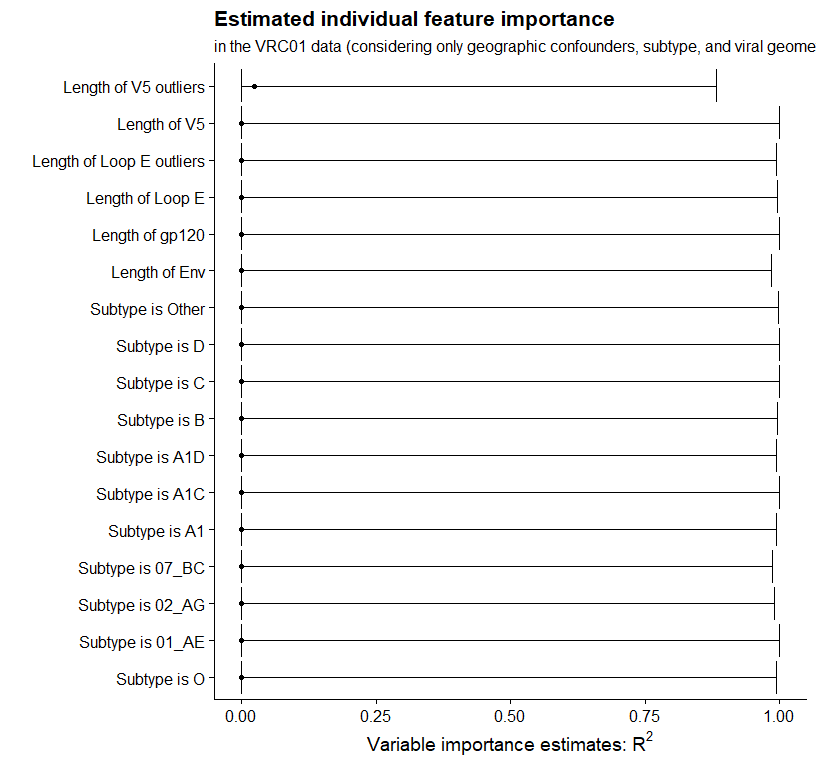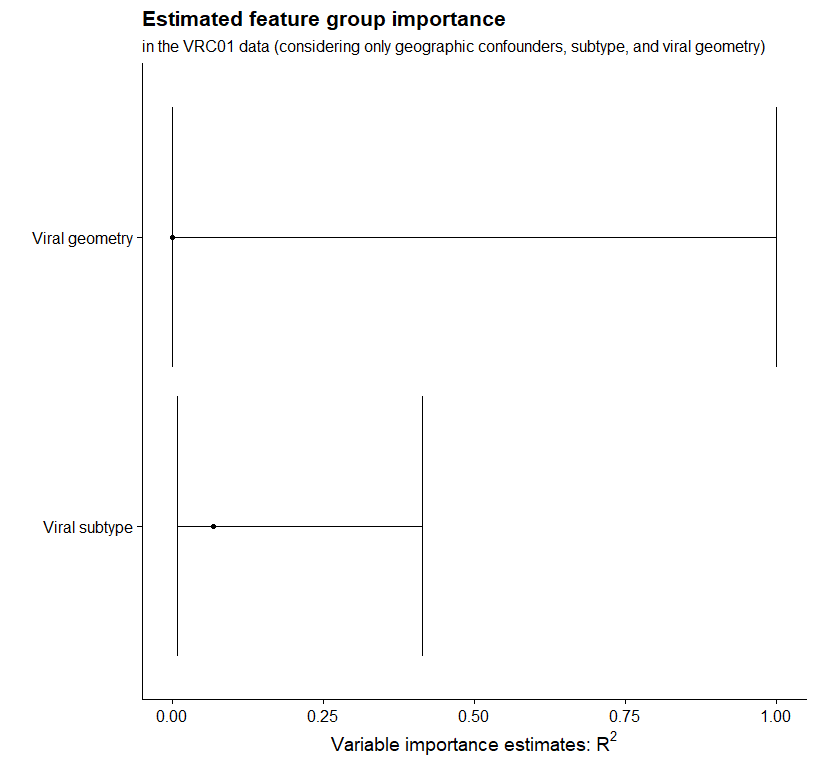# Using precomputed regression function estimates in vimp

#### 2023-08-28

library("vimp")
library("SuperLearner")

## Introduction

In the main vignette, we analyzed the VRC01 data (Magaret, Benkeser, Williamson, et al. 2019), a subset of the data freely available from the Los Alamos National Laboratory’s Compile, Neutralize, and Tally Neutralizing Antibody Panels database. Information about these data is available here. In each of the analyses, I used run_regression = TRUE. In this vignette, I discuss how to use precomputed regression function estimates with vimp. The results of this analysis replicate the analysis in the main vignette.

# read in the data
data("vrc01")
# subset to the columns of interest for this analysis
library("dplyr")
library("tidyselect")
# retain only the columns of interest for this analysis
y <- vrc01$ic50.censored X <- vrc01 %>% select(starts_with("geog"), starts_with("subtype"), starts_with("length")) set.seed(1234) vrc01_folds <- make_folds(y = y, V = 2) ## Using precomputed regression function estimates without cross-fitting ### A first approach: linear regression As in the main vignette, we first start by fitting only linear regression models. In this section, we use the function vim(); this function does not use cross-fitting to estimate variable importance, and greatly simplifies the code for precomputed regression models. library("rlang") vrc01_subset <- vrc01 %>% select(ic50.censored, starts_with("geog"), starts_with("subtype"), starts_with("length")) %>% rename(y = ic50.censored) # estimate prediction function on each subset, predict on held-out fold full_fit <- vector("numeric", length = nrow(vrc01)) for (v in 1:2) { train_v <- subset(vrc01_subset, vrc01_folds != v) test_v <- subset(vrc01_subset, vrc01_folds == v) full_mod <- glm(y ~ ., data = train_v) full_fit[vrc01_folds == v] <- predict(full_mod, newdata = test_v) } # estimate the reduced conditional means for each of the individual variables # remove the outcome for the predictor matrix geog_indx <- max(which(grepl("geog", names(X)))) for (i in seq_len(ncol(X) - geog_indx)) { this_name <- names(X)[i + geog_indx] red_fit <- vector("numeric", length = nrow(vrc01)) for (v in 1:2) { train_v <- subset(vrc01_subset, vrc01_folds != v) test_v <- subset(vrc01_subset, vrc01_folds == v) red_fit[vrc01_folds == v] <- suppressWarnings( predict(glm(y ~ ., data = train_v %>% select(-!!this_name)), newdata = test_v) ) } this_vim <- vim(Y = y, f1 = full_fit, f2 = red_fit, indx = i + geog_indx, run_regression = FALSE, type = "r_squared", sample_splitting_folds = vrc01_folds, scale = "logit") if (i == 1) { lm_mat <- this_vim } else { lm_mat <- merge_vim(lm_mat, this_vim) } } # print out the matrix lm_mat #> Variable importance estimates: #> Estimate SE 95% CI VIMP > 0 p-value #> s = 21 0.1709232 0.07644511 [0.06683172, 0.3724340] TRUE 0.01267934 #> s = 17 0.1662249 0.07767087 [0.06232601, 0.3742047] TRUE 0.01617256 #> s = 9 0.1641874 0.07765390 [0.06085506, 0.3732460] TRUE 0.01724274 #> s = 14 0.1641874 0.07765390 [0.06085506, 0.3732460] TRUE 0.01724274 #> s = 5 0.1641874 0.07765390 [0.06085506, 0.3732460] TRUE 0.01724274 #> s = 12 0.1641874 0.07765390 [0.06085506, 0.3732460] TRUE 0.01724274 #> s = 11 0.1641874 0.07765390 [0.06085506, 0.3732460] TRUE 0.01724274 #> s = 8 0.1641874 0.07765390 [0.06085506, 0.3732460] TRUE 0.01724274 #> s = 15 0.1641874 0.07765390 [0.06085506, 0.3732460] TRUE 0.01724274 #> s = 10 0.1641874 0.07765390 [0.06085506, 0.3732460] TRUE 0.01724274 #> s = 13 0.1641874 0.07765390 [0.06085506, 0.3732460] TRUE 0.01724274 #> s = 7 0.1641874 0.07765390 [0.06085506, 0.3732460] TRUE 0.01724274 #> s = 6 0.1641874 0.07765390 [0.06085506, 0.3732460] TRUE 0.01724274 #> s = 20 0.1638964 0.07774146 [0.06057232, 0.3734127] TRUE 0.01750584 #> s = 19 0.1626376 0.07934585 [0.05837835, 0.3782914] TRUE 0.02019537 #> s = 16 0.1597680 0.07730619 [0.05794103, 0.3702210] TRUE 0.01938165 #> s = 18 0.1542358 0.07595770 [0.05504322, 0.3634327] TRUE 0.02115043 ## Estimating variable importance for a single variable using precomputed regression function estimates In this section, we will use cross-fitting and pre-computed estimates of the regression functions. This can be especially useful if you have already run a call to CV.SuperLearner – that function returns estimates based on each observation being part of the hold-out set. However, while this approach can save you some computation time, it requires a hefty amount of mental overhead. We will use CV.SuperLearner to fit the individual regression functions, taking care to use the same cross-fitting folds in each regression. We will then create two groups of validation folds for sample-splitting. For this analysis, we will use V = 2 folds for cross-fitted variable importance estimation (as we did in the main vignette). Note that this entails running CV.SuperLearner with $$2V = 4$$ folds. First, we estimate the regression function based on all variables: learners <- "SL.ranger" # estimate the full regression function V <- 2 set.seed(4747) full_cv_fit <- suppressWarnings( SuperLearner::CV.SuperLearner( Y = y, X = X, SL.library = learners, cvControl = list(V = 2 * V), innerCvControl = list(list(V = V)), family = binomial() ) ) # get a numeric vector of cross-fitting folds cross_fitting_folds <- get_cv_sl_folds(full_cv_fit$folds)
# get sample splitting folds
set.seed(1234)
sample_splitting_folds <- make_folds(unique(cross_fitting_folds), V = 2)
full_cv_preds <- full_cv_fit$SL.predict Next, to estimate the importance of each variable, we need to estimate the reduced regression function for each variable: vars <- names(X)[(geog_indx + 1):ncol(X)] set.seed(1234) for (i in seq_len(length(vars))) { # use "eval" and "parse" to assign the objects of interest to avoid duplicating code eval(parse(text = paste0("reduced_", vars[i], "_cv_fit <- suppressWarnings(SuperLearner::CV.SuperLearner( Y = y, X = X[, -(geog_indx + i), drop = FALSE], SL.library = learners, cvControl = SuperLearner::SuperLearner.CV.control(V = 2 * V, validRows = full_cv_fit$folds),
innerCvControl = list(list(V = V)), family = binomial()
))")))
eval(parse(text = paste0("reduced_", vars[i], "_cv_preds <- reduced_", vars[i], "_cv_fit$SL.predict"))) } Then we can plug these values into vimp_rsquared() (or equivalently, cv_vim() with type = "r_squared") as follows: for (i in seq_len(length(vars))) { # again, use "eval" and "parse" to assign the objects of interest to avoid duplicating code eval(parse(text = paste0("cf_", vars[i], "_vim <- vimp_rsquared(Y = y, cross_fitted_f1 = full_cv_preds, cross_fitted_f2 = reduced_", vars[i], "_cv_preds, indx = (geog_indx + i), cross_fitting_folds = cross_fitting_folds, sample_splitting_folds = sample_splitting_folds, run_regression = FALSE, alpha = 0.05, V = V, na.rm = TRUE, scale = 'logit')"))) } #> Warning in cv_vim(type = "r_squared", Y = Y, X = X, cross_fitted_f1 = #> cross_fitted_f1, : Original estimate < 0; returning zero. #> Warning in cv_vim(type = "r_squared", Y = Y, X = X, cross_fitted_f1 = #> cross_fitted_f1, : Original estimate < 0; returning zero. #> Warning in cv_vim(type = "r_squared", Y = Y, X = X, cross_fitted_f1 = #> cross_fitted_f1, : Original estimate < 0; returning zero. #> Warning in cv_vim(type = "r_squared", Y = Y, X = X, cross_fitted_f1 = #> cross_fitted_f1, : Original estimate < 0; returning zero. #> Warning in cv_vim(type = "r_squared", Y = Y, X = X, cross_fitted_f1 = #> cross_fitted_f1, : Original estimate < 0; returning zero. #> Warning in cv_vim(type = "r_squared", Y = Y, X = X, cross_fitted_f1 = #> cross_fitted_f1, : Original estimate < 0; returning zero. #> Warning in cv_vim(type = "r_squared", Y = Y, X = X, cross_fitted_f1 = #> cross_fitted_f1, : Original estimate < 0; returning zero. #> Warning in cv_vim(type = "r_squared", Y = Y, X = X, cross_fitted_f1 = #> cross_fitted_f1, : Original estimate < 0; returning zero. #> Warning in cv_vim(type = "r_squared", Y = Y, X = X, cross_fitted_f1 = #> cross_fitted_f1, : Original estimate < 0; returning zero. #> Warning in cv_vim(type = "r_squared", Y = Y, X = X, cross_fitted_f1 = #> cross_fitted_f1, : Original estimate < 0; returning zero. #> Warning in cv_vim(type = "r_squared", Y = Y, X = X, cross_fitted_f1 = #> cross_fitted_f1, : Original estimate < 0; returning zero. #> Warning in cv_vim(type = "r_squared", Y = Y, X = X, cross_fitted_f1 = #> cross_fitted_f1, : Original estimate < 0; returning zero. #> Warning in cv_vim(type = "r_squared", Y = Y, X = X, cross_fitted_f1 = #> cross_fitted_f1, : Original estimate < 0; returning zero. #> Warning in cv_vim(type = "r_squared", Y = Y, X = X, cross_fitted_f1 = #> cross_fitted_f1, : Original estimate < 0; returning zero. #> Warning in cv_vim(type = "r_squared", Y = Y, X = X, cross_fitted_f1 = #> cross_fitted_f1, : Original estimate < 0; returning zero. #> Warning in cv_vim(type = "r_squared", Y = Y, X = X, cross_fitted_f1 = #> cross_fitted_f1, : Original estimate < 0; returning zero. cf_ests <- merge_vim(cf_subtype.is.01_AE_vim, cf_subtype.is.02_AG_vim, cf_subtype.is.07_BC_vim, cf_subtype.is.A1_vim, cf_subtype.is.A1C_vim, cf_subtype.is.A1D_vim, cf_subtype.is.B_vim, cf_subtype.is.C_vim, cf_subtype.is.D_vim, cf_subtype.is.O_vim, cf_subtype.is.Other_vim, cf_length.env_vim, cf_length.gp120_vim, cf_length.loop.e_vim, cf_length.loop.e.outliers_vim, cf_length.v5_vim, cf_length.v5.outliers_vim) all_vars <- c(paste0("Subtype is ", c("01_AE", "02_AG", "07_BC", "A1", "A1C", "A1D", "B", "C", "D", "O", "Other")), paste0("Length of ", c("Env", "gp120", "V5", "V5 outliers", "Loop E", "Loop E outliers"))) And we can view them all simultaneously by plotting: library("ggplot2") library("cowplot") theme_set(theme_cowplot()) cf_est_plot_tib <- cf_ests$mat %>%
mutate(
var_fct = rev(factor(s, levels = cf_ests$mat$s,
labels = all_vars[as.numeric(cf_ests$mat$s) - geog_indx],
ordered = TRUE))
)

# plot
cf_est_plot_tib %>%
ggplot(aes(x = est, y = var_fct)) +
geom_point() +
geom_errorbarh(aes(xmin = cil, xmax = ciu)) +
xlab(expression(paste("Variable importance estimates: ", R^2, sep = ""))) +
ylab("") +
ggtitle("Estimated individual feature importance") +
labs(subtitle = "in the VRC01 data (considering only geographic confounders, subtype, and viral geometry)")## Estimating variable importance for a group of variables using precomputed regression function estimates

Finally, we can estimate and plot group importance:

set.seed(91011)
reduced_subtype_cv_fit <- suppressWarnings(
SuperLearner::CV.SuperLearner(
Y = y, X = X[, -c(5:15), drop = FALSE], SL.library = learners,
cvControl = SuperLearner::SuperLearner.CV.control(V = 2 * V, validRows = full_cv_fit$folds), innerCvControl = list(list(V = V)), family = binomial() ) ) reduced_subtype_cv_preds <- reduced_subtype_cv_fit$SL.predict
reduced_geometry_cv_fit <- suppressWarnings(
SuperLearner::CV.SuperLearner(
Y = y, X = X[, -c(16:21), drop = FALSE], SL.library = learners,
cvControl = SuperLearner::SuperLearner.CV.control(V = 2 * V, validRows = full_cv_fit$folds), innerCvControl = list(list(V = V)), family = binomial() ) ) reduced_geometry_cv_preds <- reduced_geometry_cv_fit$SL.predict
cf_subtype_vim <- vimp_rsquared(
Y = y, cross_fitted_f1 = full_cv_preds, cross_fitted_f2 = reduced_subtype_cv_preds,
indx = 5:15, run_regression = FALSE, V = V,
cross_fitting_folds = cross_fitting_folds, sample_splitting_folds = sample_splitting_folds,
scale = "logit"
)
cf_geometry_vim <- vimp_rsquared(
Y = y, cross_fitted_f1 = full_cv_preds, cross_fitted_f2 = reduced_geometry_cv_preds,
indx = 16:21, run_regression = FALSE, V = V,
cross_fitting_folds = cross_fitting_folds, sample_splitting_folds = sample_splitting_folds,
scale = "logit"
)
cf_groups <- merge_vim(cf_subtype_vim, cf_geometry_vim)
all_grp_nms <- c("Viral subtype", "Viral geometry")

grp_plot_tib <- cf_groups\$mat %>%
mutate(
grp_fct = factor(case_when(
s == "5,6,7,8,9,10,11,12,13,14,15" ~ "1",
s == "16,17,18,19,20,21" ~ "2"
), levels = c("1", "2"),  labels = all_grp_nms, ordered = TRUE)
)
grp_plot_tib %>%
ggplot(aes(x = est, y = grp_fct)) +
geom_point() +
geom_errorbarh(aes(xmin = cil, xmax = ciu)) +
xlab(expression(paste("Variable importance estimates: ", R^2, sep = ""))) +
ylab("") +
ggtitle("Estimated feature group importance") +
labs(subtitle = "in the VRC01 data (considering only geographic confounders, subtype, and viral geometry)")## Conclusion

In this document, we learned a second method for computing variable importance estimates: rather than having vimp run all regression functions for you, you can compute your own regressions and pass these to vimp. The results are equivalent, but there is a tradeoff: what you save in computation time by only computing the full regression once must be balanced with the mental overhead of correctly computing the regressions. Additionally, this task is more difficult when using cross-fitted variable importance, which I recommend in nearly all cases when using flexible machine learning tools.